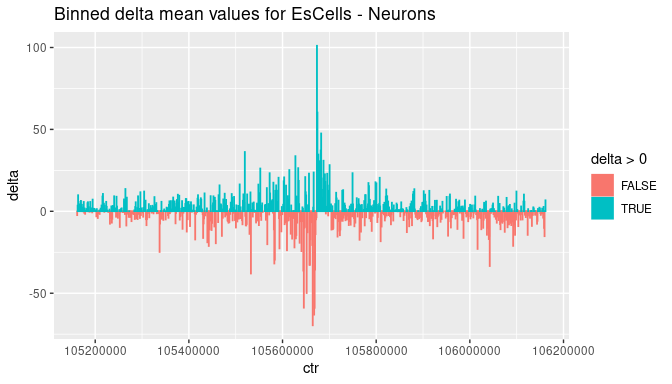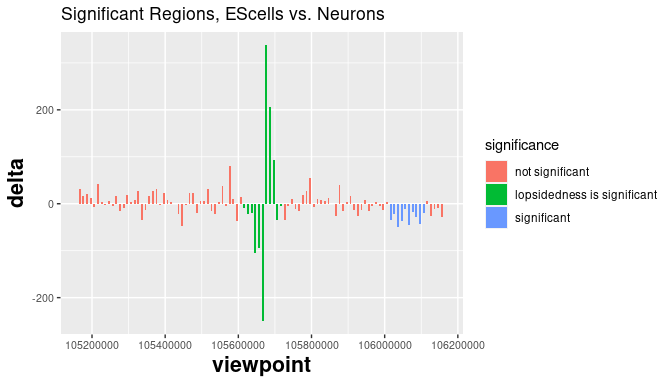# deltaCaptureC

## Introduction

The purpose of this package is to detect meso-scale changes in chromatin conformation from 3C data. Typical 3C data might look like the following:

chr start end EScells_1
chr2 13505797 13506141 2
chr2 13506144 13506556 2
chr2 13654334 13655871 14
chr2 105656443 105656693 241
chr2 105656696 105659412 263
chr2 105659415 105660479 126
chr2 105662321 105663389 275
chr2 105663392 105663974 615
chr2 173656857 173657083 2
chr2 173694707 173695349 2
chr2 173698231 173698911 4

The segments shown are restriction enzyme digestion fragments. The counts show the numbers of experimentally captured interactions captured interactions between each of these fragments and the Paupar viewpoint. This viewpoint lies in the region 105661048 -105661864 and we see higher numbers of captures for digestion fragments near the viewpoint. Packages like r3Cseq attempt to determine p-values for the numbers of captures for each fragment. In order to do this, it estimates a ‘background’ level of interaction as a function of distance from the viewpoint. Comparing the count for each fragment to this background level allows it to estimate significance for each fragment.

This method makes only limited use of positional information. While it considers distance from the viewpoint in estimating background levels it ignores the proximity of individual fragments. But suppose we have ten consecutive fragments, each with counts only slightly above background. None may rise to statistical significance on its own, but their co-location may make the group highly significant. We will exploit this observation to look for statistically significant changes in counts between treatments or cell types.

Consider 3C data for the same viewpoint for two replicates each for two different cell types.

chr start end EScells_1 EScells_2 Neu_1 Neu_2
chr2 6226506 6226673 0 2 0 0
chr2 6235906 6237082 0 0 0 1
chr2 6270043 6270850 1 0 0 0
chr2 105656443 105656693 241 120 184 82
chr2 105656696 105659412 263 215 365 225
chr2 105659415 105660479 126 182 220 160
chr2 105662321 105663389 275 90 171 133
chr2 105663392 105663974 615 166 327 301
chr2 179455636 179455885 0 0 0 1
chr2 179473020 179473517 0 0 0 3
chr2 179473520 179473584 0 0 0 3

We wish to discover regions where there is a statisitcally significant change in the interaction levels between the two cell types.

## Difference in mean normalized counts:

The first task is to normalize the values for each of the cell types. This will allow us to find the mean count for each of the digestion fragments in each of the cell types and consequently find the difference in the mean normalized counts for the two cell types. Normalizing depends on estimating a size factor for each replicate. Here we are relying on DESeq2::estimateSizeFactorsForMatrix(). For further details see the DESeq2 vignette or Love, M.I., Huber, W., Anders, S. Moderated estimation of fold change and dispersion for RNA-seq data with DESeq2 Genome Biology 15(12):550 (2014)

We have included a miniature version of the summarized experiment miniSE in order to demonstrate the process of extracting the delta mean normalized value miniDeltaSE.

{r}
library(deltaCaptureC)
miniDeltaSE = getDeltaSE(miniSE)

The actual deltaSE comes from a SummarizedExperiment representing the complete data set for these four replicates and is subsequently trimmed to a region on chromosome2 surrounding our region of interest. After binning to a bin size of 1kb it looks like this:Our interface carries out the binning inside the call to the function getSignificantRegions(). The delta data (here deltaSE) is then mined for significant regions.

## The Algorithm

The algorithm proceeds as follows:

1. The data is binned to smallBinSize (here 1kb) and trimmed to the region of interest. The region of interest here is 500kb up and downstream of the mid-point of the viewpoint.
2. We now rebin this data to bigBinSize (here 10kb). This value should be an integer multiple of smallBinSize.
3. The interaction count data are highest near the viewpoint. We therefore distinguish a viewpoint region.
• In the viewpoint region, we compute the lopsidedness of the data, i.e., the absolute value of the difference between the sum of the bins to the left of the viewpoint and the sum of the bins to the right of the viewpoint.
• Outside the viewpoint region we define runs to be maximal consecutive sequences of bins where the delta value does not change sign. We compute the totals of the bins in each run. We call these run totals.
4. We now perform random permutations of the small-bin delta data.
• Within the viewpoint region, these permutations respect the distance from the viewpoint. Thus, for each distance k from the viewpoint, the two bins at distance k either are or are not swapped.
• We perform arbitrary permutations on the non-viewpoint bins.
5. For each permutation, we rebin the resulting scrambled data to the large bin size and then compute the lopsidedness and run totals for that permutation.
6. For each permutation we record the lopsidedness and maximum and minimum of the run totals. Across all permutations this produces
• a distribution of lopsidedness values
• a distribution of maximum run totals
• a distribution of minimum run totals
7. Significance in the original (unpermuted) data is then assessed by comparing the lopsidedness and run totals in the original data to these distributions.

## Invoking the algorithm and plotting the results

significantRegions = getSignificantRegions(deltaSE,
regionOfInterest,
viewpointRegion,
smallBinSize,
bigBinSize,
numPermutations,
pValue)

which are then ploted using

library(deltaCaptureC)
significantRegionsPlot = plotSignificantRegions(significantRegions,
significanceType,
plotTitle)

This gives the following result:## Under the hood

### Normalization

We have given an example above of the use of getDeltaSE(), converting miniSE into miniDeltaSE. This is a multi-step process, first normalizing the columns of miniSE, then averaging the values for each of the two treatments and finally taking their difference. For normalization, we relied on DESeq2::estimateSizeFactorsForMatrix().

library(SummarizedExperiment)
library(deltaCaptureC)
se = miniSE
counts = assays(se)[['counts']]
sizeFactors = DESeq2::estimateSizeFactorsForMatrix(counts)
colData(se)$sizeFactors = sizeFactors assays(se)[['normalizedCounts']] = counts for(i in seq_len(ncol(assays(se)[['normalizedCounts']]))) { assays(se)[['normalizedCounts']][,i] = assays(se)[['normalizedCounts']][,i] / colData(se)$sizeFactors[i]
}

Depending on your application, you may wish to use your own method of normalization.

The delta SummarizedExperiment is then the difference between the mean expressions for the two treatments.

library(SummarizedExperiment)
library(deltaCaptureC)
meanNormalizedCountsSE = getMeanNormalizedCountsSE(miniSE)
meanCounts = assay(meanNormalizedCountsSE)
delta = matrix(meanCounts[,1] - meanCounts[,2],ncol=1)
colData = data.frame(delta=sprintf('%s - %s',
as.character(colData(meanNormalizedCountsSE)$treatment), as.character(colData(meanNormalizedCountsSE)$treatment)),
stringsAsFactors=FALSE)
deltaSE = SummarizedExperiment(assay=list(delta=delta),
colData=colData)
rowRanges(deltaSE) = rowRanges(meanNormalizedCountsSE)

Binning

Binning is the rate-limiting step as can be seen from the binning of a small Summarized experiment into a small set of bins:

library(deltaCaptureC)
print(length(smallSetOfSmallBins))
#>  201
print(length(smallerDeltaSE))
#>  401
tictoc::tic('binning into small bins')
binnedSummarizedExperiment = binSummarizedExperiment(smallSetOfSmallBins,smallerDeltaSE)
tictoc::toc()
#> binning into small bins: 101.17 sec elapsed

The algorithm depends on the permutation of small bins and the rebinning of the resulting permuted data into large bins for comparison to the actual data binned into the same larger bins. The binning into smaller bins only happens once, while the rebinning into larger bins is carried out for each permutation. Consequently, we require the larger bin size to be an integer multiple of the smaller and this rebinning is much faster.

library(deltaCaptureC)
tictoc::tic('rebinning to larger bin size')
rebinnedSummarizedExperiment =
rebinToMultiple(binnedSummarizedExperiment,10)
tictoc::toc()
#> rebinning to larger bin size: 0.208 sec elapsed